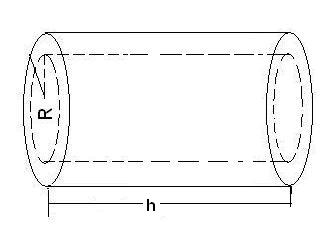### Calculate Volume of Pipe or Tube

Calculate the cylindrical volume of a tube, also called a pipe or hollow cylinder for given values using following formula :
cylindrical volume (V) = π(r12 - r22 )h
where, r1 = Outer radius, r2 = inner radius and h = heightOuter radius Inner radius Height Cylindrical Volume